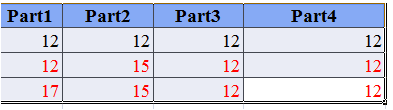# How to give red color for rows on html table that have different values on same row?

How to give red color for rows on html table that have different values on same row ?

I work on dynamic table not static

I need when at least one cell from row different from each others

then give red font to full row

if you see below row that have same value on all cell not changed color .

I need function give red color for row that have at least one value different from others on same row

so How to make function do that by jquery or java script do red color for row if it have

at least different cell value .

rows have different values give red rows font``````<!DOCTYPE html>
<html>
<body>
<table border="1">
<col width="500">
<col width="500">
<col width="500">
<col width="500">
<tr bgcolor="#6699FF" width="100%">
<th>Part1</th>
<th>Part2</th>
<th>Part3</th>
<th>Part4</th>
<tr>
<td>12</td>
<td>12</td>
<td>12</td>
<td>12</td>
</tr>
<tr>
<td>12</td>
<td>15</td>
<td>12</td>
<td>12</td>
</tr>
<tr>
<td>17</td>
<td>15</td>
<td>13</td>
<td>12</td>
</tr>
</table>

<button id="button">Click Me</button>
</body>
``````

I assume the data comes in array, so you could check first if all the data is the same value or not.
If not, then when creating row detail (td) for each data in that array, you can add the class like `.red-number`
And add a CSS rule for that class.

``````.red-number {
color: "red";
}
``````

can you give me more details if possible

you can create a table with two colors using javascript as follows:

``````<!DOCTYPE html>
<html>
<body>
<table id="table" border="1">
<col width="500">
<col width="500">
<col width="500">
<col width="500">
<tr bgcolor="#6699FF" width="100%">
<th>Part1</th>
<th>Part2</th>
<th>Part3</th>
<th>Part4</th>
</tr>
</table>
<button id="button">Click Me</button>
<script>
function check(arr){
var val=[];
for(var i=0;i<arr.length;i++){
if(arr[i]==arr[i+1] && i!=arr.length-1){
val.push(arr[i]);
}
}
if(val.length!=arr.length-1){
return "red";
}else{
return "black";
}
}
var elements="";
var array=[[12,12,12,12],[12,15,12,12],[17,15,13,12]];
for(var i=0;i<array.length;i++){
elements=elements+'<tr style="color:'+check(array[i])+';">';
for(var j=0;j<array[i].length;j++){
elements=elements+"<td>"+array[i][j]+"</td>"
}
elements=elements+"</tr>";
}
var html=document.getElementById('table').innerHTML;
document.getElementById('table').innerHTML=html+elements;
</script>
</body>
</html>
``````